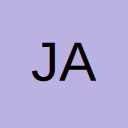# Spawning in multiple items using the events.xml and cfgeventspawns.xml files

Nitrado now has an official Discord server to bring communities, friends and other gamers together!
Join the Nitrado Community Discord now and share your experiences and knowledge with others!

• First it has to be said that it would be super nice if excuses weren't maintained about why we (the admins trying to keep DayZ alive on PS and Xbox) aren't given real admin abilities and access to ALL the files. Seriously Nitrado I know terms have been determined by the game developer but no one is going to want to host these for console knowing full well what we have virtually no real-time admin abilities in comparison to PC server DayZ admins.

Ok so on with this issue. I need to spawn in full gear sets with weapons only in specific parts of the map for a death match server and I've tried individual events as well one event with multiple child events with no success beyond the first item in a list of about 34 spawning in. So I imagine multiple items cannot be on the exact same coordinate. My issue with trying to use a container coded for cargo is that I'm pretty sure that means that container (seachest, truck, barrel) will spawn that same stuff everywhere it spawns on the map. Has anyone else worked through this multipoint issue to achieve certain items spawning in in a set location after server start and events kick off for let's say an hour and then despawning in say an hour?

• Here is the code I've tried:

cfgeventspawns.xml (method1)

<event name="Item_DeathMatch_Gear">

<pos x="13031.9" z="10572.9" a="113.000000" />

</event>

events.xml (method 1)

<event name="Item_DeathMatch_Gear">

<nominal>1</nominal>

<min>1</min>

<max>1</max>

<restock>0</restock>

<flags deletable="0" init_random="0" remove_damaged="0"/>

<position>fixed</position>

<limit>mixed</limit>

<active>1</active>

<children>

<child lootmax="0" lootmin="0" max="1" min="30" type="GhillieHood_Woodland"/>

<child lootmax="0" lootmin="0" max="1" min="30" type="GhillieSuit_Woodland"/>

<child lootmax="0" lootmin="0" max="1" min="30" type="NVGHeadstrap"/>

<child lootmax="0" lootmin="0" max="1" min="30" type="NVGoggles"/>

<child lootmax="0" lootmin="0" max="1" min="30" type="Battery9V"/>

<child lootmax="0" lootmin="0" max="1" min="30" type="BalaclavaMask_Black"/>

<child lootmax="0" lootmin="0" max="1" min="30" type="BDUJacket"/>

<child lootmax="0" lootmin="0" max="1" min="30" type="BDUPants"/>

<child lootmax="0" lootmin="0" max="1" min="30" type="PlateCarrierVest"/>

<child lootmax="0" lootmin="0" max="1" min="30" type="PlateCarrierPouches"/>

<child lootmax="0" lootmin="0" max="1" min="30" type="PlateCarrierHolster"/>

<child lootmax="0" lootmin="0" max="1" min="30" type="AliceBag_Camo"/>

<child lootmax="0" lootmin="0" max="1" min="30" type="MilitaryBelt"/>

<child lootmax="0" lootmin="0" max="1" min="30" type="NylonKnifeSheath"/>

<child lootmax="0" lootmin="0" max="1" min="30" type="TacticalGloves_Green"/>

<child lootmax="0" lootmin="0" max="1" min="30" type="ItemMilitaryBoots_Brown"/>

<child lootmax="0" lootmin="0" max="1" min="30" type="Compass"/>

<child lootmax="0" lootmin="0" max="1" min="30" type="PetrolLighter"/>

<child lootmax="0" lootmin="0" max="1" min="30" type="PeachesCan"/>

<child lootmax="0" lootmin="0" max="1" min="30" type="AKM"/>

<child lootmax="0" lootmin="0" max="1" min="30" type="FNX45"/>

<child lootmax="0" lootmin="0" max="1" min="30" type="CombatKnife"/>

<child lootmax="0" lootmin="0" max="1" min="30" type="M67Grenade"/>

<child lootmax="0" lootmin="0" max="1" min="30" type="AmmoBox_308Win_20Rnd"/>

<child lootmax="0" lootmin="0" max="1" min="30" type="AmmoBox_762x39_20Rnd"/>

<child lootmax="0" lootmin="0" max="1" min="30" type="AmmoBox_45ACP_25rnd"/>

<child lootmax="0" lootmin="0" max="1" min="30" type="WoodAxe"/>

<child lootmax="0" lootmin="0" max="1" min="30" type="Winchester70"/>

<child lootmax="0" lootmin="0" max="1" min="30" type="HuntingOptic"/>

<child lootmax="0" lootmin="0" max="1" min="30" type="TetracyclineAntibiotics"/>

<child lootmax="0" lootmin="0" max="1" min="30" type="VitaminBottle"/>

<child lootmax="0" lootmin="0" max="1" min="30" type="BandageDressing"/>

</children

</event>

• also tried this in events.xml (method 2) note on my actual server the indents are actually there

<event name="ItemGhillieSuit_Woodland">

<nominal>1</nominal>

<min>1</min>

<max>1</max>

<restock>0</restock>

<flags deletable="0" init_random="0" remove_damaged="0"/>

<position>fixed</position>

<limit>mixed</limit>

<active>1</active>

<children>

<child lootmax="0" lootmin="0" max="1" min="1" type="GhillieSuit_Woodland"/>

</children>

</event>

<nominal>1</nominal>

<min>1</min>

<max>1</max>

<restock>0</restock>

<flags deletable="0" init_random="0" remove_damaged="0"/>

<position>fixed</position>

<limit>mixed</limit>

<active>1</active>

<children>

<child lootmax="0" lootmin="0" max="1" min="1" type="NVGHeadstrap"/>

</children>

</event>

<event name="ItemNVGoggles">

<nominal>1</nominal>

<min>1</min>

<max>1</max>

<restock>0</restock>

<flags deletable="0" init_random="0" remove_damaged="0"/>

<position>fixed</position>

<limit>mixed</limit>

<active>1</active>

<children>

<child lootmax="0" lootmin="0" max="1" min="1" type="NVGoggles"/>

</children>

</event>

<event name="ItemBattery9V">

<nominal>1</nominal>

<min>1</min>

<max>1</max>

<restock>0</restock>

<flags deletable="0" init_random="0" remove_damaged="0"/>

<position>fixed</position>

<limit>mixed</limit>

<active>1</active>

<children>

<child lootmax="0" lootmin="0" max="1" min="1" type="Battery9V"/>

</children>

</event>

<nominal>1</nominal>

<min>1</min>

<max>1</max>

<restock>0</restock>

<flags deletable="0" init_random="0" remove_damaged="0"/>

<position>fixed</position>

<limit>mixed</limit>

<active>1</active>

<children>

<child lootmax="0" lootmin="0" max="1" min="1" type="BalaclavaMask_Black"/>

</children>

</event>

<event name="ItemBDUJacket">

<nominal>1</nominal>

<min>1</min>

<max>1</max>

<restock>0</restock>

<flags deletable="0" init_random="0" remove_damaged="0"/>

<position>fixed</position>

<limit>mixed</limit>

<active>1</active>

<children>

<child lootmax="0" lootmin="0" max="1" min="1" type="BDUJacket"/>

</children>

</event>

<event name="ItemBDUPants">

<nominal>1</nominal>

<min>1</min>

<max>1</max>

<restock>0</restock>

<flags deletable="0" init_random="0" remove_damaged="0"/>

<position>fixed</position>

<limit>mixed</limit>

<active>1</active>

<children>

<child lootmax="0" lootmin="0" max="1" min="1" type="BDUPants"/>

</children>

</event>

<event name="ItemPlateCarrierVest">

<nominal>1</nominal>

<min>1</min>

<max>1</max>

<restock>0</restock>

<flags deletable="0" init_random="0" remove_damaged="0"/>

<position>fixed</position>

<limit>mixed</limit>

<active>1</active>

<children>

<child lootmax="0" lootmin="0" max="1" min="1" type="PlateCarrierVest"/>

</children>

</event>

<event name="ItemPlateCarrierPouches">

<nominal>1</nominal>

<min>1</min>

<max>1</max>

<restock>0</restock>

<flags deletable="0" init_random="0" remove_damaged="0"/>

<position>fixed</position>

<limit>mixed</limit>

<active>1</active>

<children>

<child lootmax="0" lootmin="0" max="1" min="1" type="PlateCarrierPouches"/>

</children>

</event>

<event name="ItemPlateCarrierHolster">

<nominal>1</nominal>

<min>1</min>

<max>1</max>

<restock>0</restock>

<flags deletable="0" init_random="0" remove_damaged="0"/>

<position>fixed</position>

<limit>mixed</limit>

<active>1</active>

<children>

<child lootmax="0" lootmin="0" max="1" min="1" type="PlateCarrierHolster"/>

</children>

</event>

<event name="ItemAliceBag_Camo">

<nominal>1</nominal>

<min>1</min>

<max>1</max>

<restock>0</restock>

<flags deletable="0" init_random="0" remove_damaged="0"/>

<position>fixed</position>

<limit>mixed</limit>

<active>1</active>

<children>

<child lootmax="0" lootmin="0" max="1" min="1" type="AliceBag_Camo"/>

</children>

</event>

<event name="ItemMilitaryBelt">

<nominal>1</nominal>

<min>1</min>

<max>1</max>

<restock>0</restock>

<flags deletable="0" init_random="0" remove_damaged="0"/>

<position>fixed</position>

<limit>mixed</limit>

<active>1</active>

<children>

<child lootmax="0" lootmin="0" max="1" min="1" type="MilitaryBelt"/>

</children>

</event>

<event name="ItemNylonKnifeSheath">

<nominal>1</nominal>

<min>1</min>

<max>1</max>

<restock>0</restock>

<flags deletable="0" init_random="0" remove_damaged="0"/>

<position>fixed</position>

<limit>mixed</limit>

<active>1</active>

<children>

<child lootmax="0" lootmin="0" max="1" min="1" type="NylonKnifeSheath"/>

</children>

</event>

<event name="ItemTacticalGloves_Green">

<nominal>1</nominal>

<min>1</min>

<max>1</max>

<restock>0</restock>

<flags deletable="0" init_random="0" remove_damaged="0"/>

<position>fixed</position>

<limit>mixed</limit>

<active>1</active>

<children>

<child lootmax="0" lootmin="0" max="1" min="1" type="TacticalGloves_Green"/>

</children>

</event>

<event name="ItemMilitaryBoots_Brown">

<nominal>1</nominal>

<min>1</min>

<max>1</max>

<restock>0</restock>

<flags deletable="0" init_random="0" remove_damaged="0"/>

<position>fixed</position>

<limit>mixed</limit>

<active>1</active>

<children>

<child lootmax="0" lootmin="0" max="1" min="1" type="MilitaryBoots_Brown"/>

</children>

</event>

cfgeventspawns.xml (method2)

<event name="ItemGhillieHood_Woodland">

<pos x="12944.7" z="10616.3" a="113.000000" />

</event>

<event name="ItemGhillieSuit_Woodland">

<pos x="12944.7" z="10616.3" a="113.000000" />

</event>

<pos x="12944.7" z="10616.3" a="113.000000" />

</event>

<event name="ItemNVGoggles">

<pos x="12944.7" z="10616.3" a="113.000000" />

</event>

<event name="ItemBattery9V">

<pos x="12944.7" z="10616.3" a="113.000000" />

</event>

<pos x="12944.7" z="10616.3" a="113.000000" />

</event>

<event name="ItemBDUJacket">

<pos x="12944.7" z="10616.3" a="113.000000" />

</event>

<event name="ItemBDUPants">

<pos x="12944.7" z="10616.3" a="113.000000" />

</event>

<event name="ItemPlateCarrierVest">

<pos x="12944.7" z="10616.3" a="113.000000" />

</event>

<event name="ItemPlateCarrierPouches">

<pos x="12944.7" z="10616.3" a="113.000000" />

</event>

<event name="ItemPlateCarrierHolster">

<pos x="12944.7" z="10616.3" a="113.000000" />

</event>

<event name="ItemAliceBag_Camo">

<pos x="12944.7" z="10616.3" a="113.000000" />

</event>

<event name="ItemMilitaryBelt">

<pos x="12944.7" z="10616.3" a="113.000000" />

</event>

<event name="ItemNylonKnifeSheath">

<pos x="12944.7" z="10616.3" a="113.000000" />

</event>

<event name="ItemTacticalGloves_Green">

<pos x="12944.7" z="10616.3" a="113.000000" />

</event>

<event name="ItemMilitaryBoots_Brown">

<pos x="12944.7" z="10616.3" a="113.000000" />

</event>

• I've rented a second server as a staging branch to test this...come on do no admins spawn stuff into the game as part of admin work?